The core math topics covered in grade 3 typically are:

Numbers and Operations

• adding and subtracting within 1,000
• place value of ones, tens and hundreds
• rounding numbers to the nearest 10 or 100
• relationship between addition and subtraction
• introduction to multiplication
• introduction to division
• multiply and divide within 100
• fractions as parts of a whole

Geometry

• classification of shapes by their properties
• partitioning of shapes into equal parts
• area and perimeter of irregular shapes by counting squares
• area and perimeter of rectangles

Measurement

• estimating and measuring masses
• measuring lengths (including in fractions of an inch)
• estimating and measuring volumes of liquids
• telling time to the nearest minute and time word problems

Data Analysis

• Picture graphs and bar graphs to represent data in multiple categories
• Line plots

You may also want to read the common core math standards for grade 3.

## Math worksheets for grade 3

Choose from hundreds of free grade 3 math worksheets organized by topic:

 Place Value and Rounding Fractions and Decimals Order of Operations Measurement Addition Counting Money Subtraction Time & Calendar Multiplication Geometry Division Word Problems Roman Numerals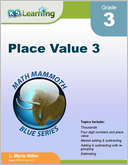Place Value 3 Four digit numbers; place values of ones, tens, hundreds and thousands.  Topics include comparing numbers, adding and subtracting with regrouping, rounding, estimating and word problems.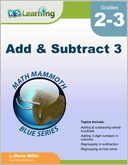Add & Subtract 3 Adding and subtracting 3-digit numbers, mentally and in columns, within 1-1,000. Includes regrouping tens as hundreds and regrouping across zeros. Also ordinal numbers, roman numerals and order of operations.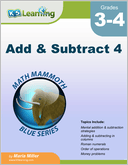Add and Subtract 4 Mental addition and subtraction strategies; adding and subtracting in columns and order of operations.  Also Roman numerals, money problems and bar and line graphs.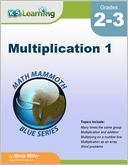Multiplication 1 Introducing the concept of multiplication and the order of operations.  The second part of the book focuses on the memorization of the multiplication tables.  Includes word problems.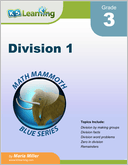Division 1 Introducing division, in particular single digit division, remainders and divisibility.  Division by making groups, division facts and division word problems.  Remainders and divisibility rules.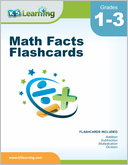Math Facts Flashcards Flashcards for addition, subtraction, multiplication and division facts, 0-12.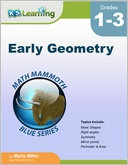Early Geometry Basic shapes (rectangles, squares,...), right angles, symmetry, mirror points, perimeter and area.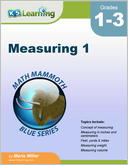Measuring 1 Length, weight and capacity for grades 1-3, including the concept of measuring lengths, weights and volumes.  Both customary and metric systems are used.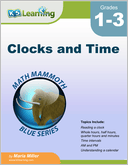Reading a clock, time intervals, am & pm and understanding a calendar.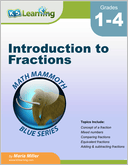Introduction to Fractions Introducing fractions, mostly for grades 3-4. Concept of a fraction, mixed numbers, comparing fractions, equivalent fractions, adding and subtracting fractions and fractions multiplied by a whole number.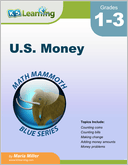U.S. Money Recognizing coins and bills; counting money, making change and money problems. We also have similar books in other currencies (Canadian money, Australian Money, British Money, European Money and South African Money).